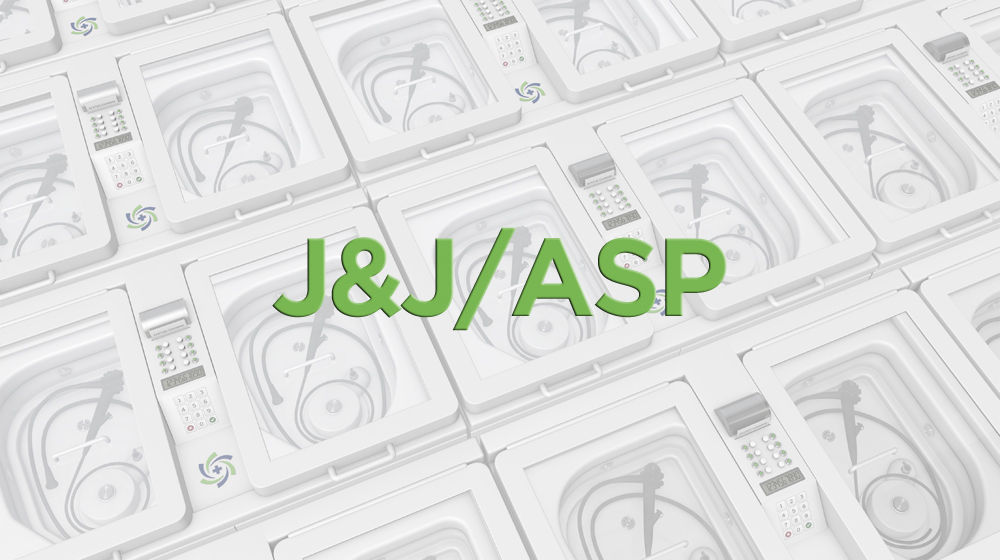( Case of 50 )

( Each )

( Each )

( Each )

( Each )

Free

( Each )

( Case of 8 )

( Each )

( Each )

( Each )

( Each )

( 4gal / case )

( 100 Sponges )

( 36 Kits/case )

( Case of 12 )

Free

( Case of 12 )

( Each )

( Each )

( Each )

( Each )

( 24 Kits/case )

( Combo Pack )

( Each )

Free

Free

( Each )

( Each )

( Each )

( Each )

( Each )

( Case of 50 )

( 312 Tablets )

( Combo Pack )

( Each )

( Each )

( Each )

( Each )

( Each )

( 4gal / case )

Free

( Each )

( Each )

( 10 Rolls )

( 100 Strips )

Free

Free

( Each )

( Case of 6 )

( Case of 8 )

( Each )

( 4gal / case )

( Each )

( 4gal / case )

( 1 )

( 5 Rolls )

Free

Free

( Individually Pkgd / Case of 100 )

( 4gal / case )

( 200 Strips )

Results 1 - 120 of 147